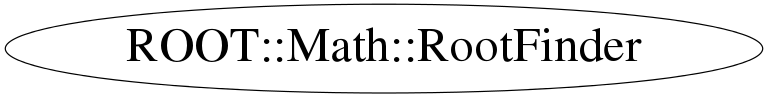# class ROOT::Math::RootFinder

```
User Class to find the Root of one dimensional functions.
The GSL Methods are implemented in MathMore and they are loaded automatically
via the plug-in manager

The possible types of Root-finding algorithms are:
<ul>
<li>Root Bracketing Algorithms which do not require function derivatives
<ol>
<li>RootFinder::kBRENT  (default method implemented in MathCore)
<li>RootFinder::kGSL_BISECTION
<li>RootFinder::kGSL_FALSE_POS
<li>RootFinder::kGSL_BRENT
</ol>
<li>Root Finding Algorithms using Derivatives
<ol>
<li>RootFinder::kGSL_NEWTON
<li>RootFinder::kGSL_SECANT
<li>RootFinder::kGSL_STEFFENSON
</ol>
</ul>

This class does not cupport copying

@ingroup RootFinders

```

## Function Members (Methods)

public:
 virtual ~RootFinder() int Iterate() int Iterations() const const char* Name() const double Root() const ROOT::Math::RootFinder RootFinder(ROOT::Math::RootFinder::EType type = RootFinder::kBRENT) bool SetFunction(const ROOT::Math::IGradFunction& f, double xstart) bool SetFunction(const ROOT::Math::IGenFunction& f, double xlow, double xup) bool SetMethod(ROOT::Math::RootFinder::EType type = RootFinder::kBRENT) bool Solve(int maxIter = 100, double absTol = 1.0E-8, double relTol = 1.0E-10) bool Solve(int& f, double& d, double start, int maxIter = 100, double absTol = 1.0E-8, double relTol = 1.0E-10) bool Solve(int& f, double min, double max, int maxIter = 100, double absTol = 1.0E-8, double relTol = 1.0E-10) int Status() const
private:
 ROOT::Math::RootFinder& operator=(const ROOT::Math::RootFinder& rhs) ROOT::Math::RootFinder RootFinder(const ROOT::Math::RootFinder&)

## Data Members

public:
 static ROOT::Math::RootFinder::EType kBRENT static ROOT::Math::RootFinder::EType kGSL_BISECTION static ROOT::Math::RootFinder::EType kGSL_BRENT static ROOT::Math::RootFinder::EType kGSL_FALSE_POS static ROOT::Math::RootFinder::EType kGSL_NEWTON static ROOT::Math::RootFinder::EType kGSL_SECANT static ROOT::Math::RootFinder::EType kGSL_STEFFENSON
private:
 ROOT::Math::IRootFinderMethod* fSolver type of algorithm to be used

## Class Charts## Function documentation

bool Solve(Function &f, Derivative &d, double start, int maxIter, double absTol, double relTol)
RootFinder(RootFinder::EType type = RootFinder::kBRENT)
```            Construct a Root-Finder algorithm

```
virtual ~RootFinder()
RootFinder(const RootFinder & )
``` usually copying is non trivial, so we make this unaccessible
```
`{}`
bool SetMethod(ROOT::Math::RootFinder::EType type = RootFinder::kBRENT)
bool SetFunction(const ROOT::Math::IGenFunction& f, double xlow, double xup)
```            Provide to the solver the function and the initial search interval [xlow, xup]
for algorithms not using derivatives (bracketing algorithms)
The templated function f must be of a type implementing the \a operator() method,
<em>  double  operator() (  double  x ) </em>
Returns non zero if interval is not valid (i.e. does not contains a root)

```
return fSolver-> SetFunction(const ROOT::Math::IGenFunction& f, double xlow, double xup)
int Iterations() const
```             Return the number of iteration performed to find the Root.

```
int Iterate()
```            Perform a single iteration and return the Status

```
double Root() const
```            Return the current and latest estimate of the Root

```
int Status() const
```            Return the status of the last estimate of the Root
= 0 OK, not zero failure

```
const char * Name() const
```            Return the current and latest estimate of the lower value of the Root-finding interval (for bracketing algorithms)

double XLower() const {
return fSolver->XLower();
}

Return the current and latest estimate of the upper value of the Root-finding interval (for bracketing algorithms)

double XUpper() const {
return  fSolver->XUpper();
}

Get Name of the Root-finding solver algorithm

```